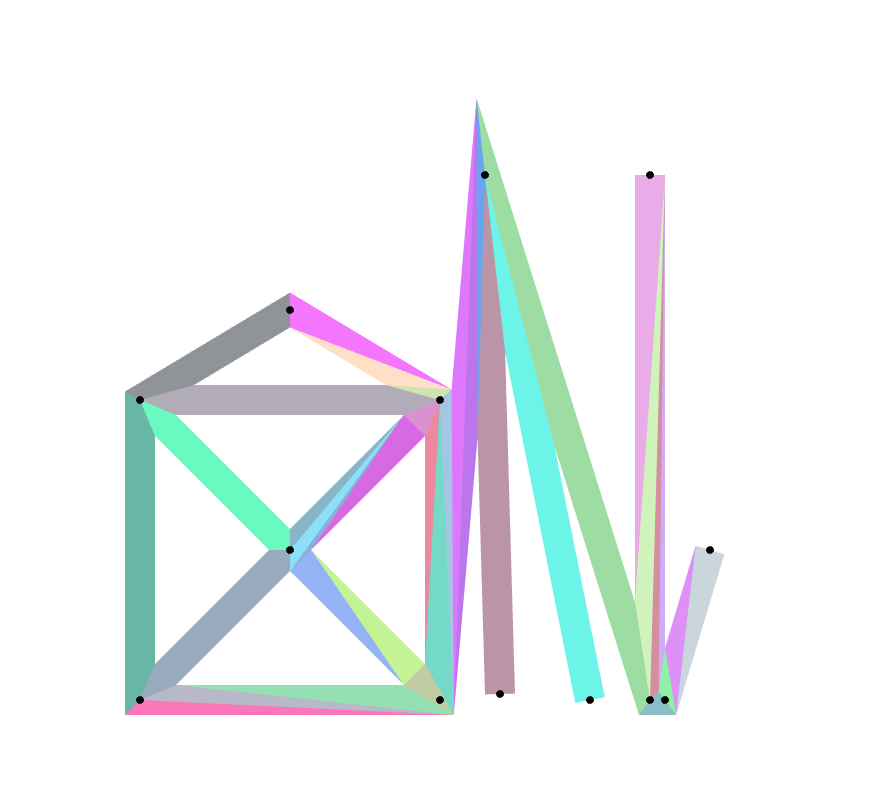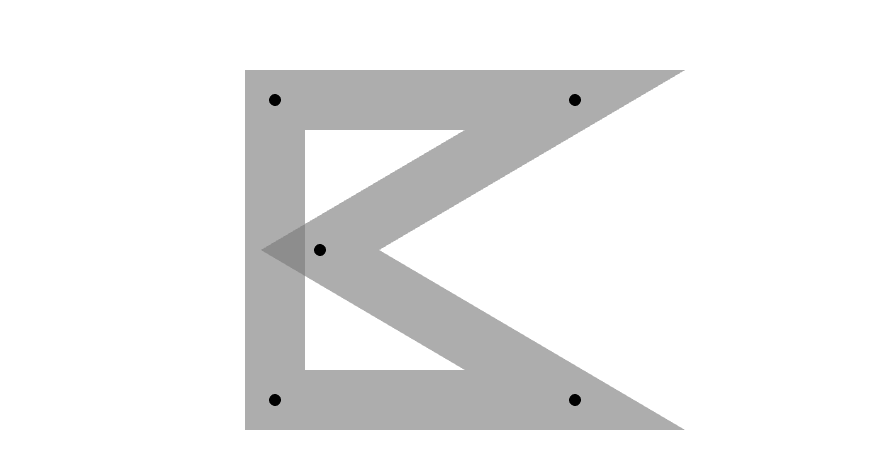Nerdy People Matriculate

# npm

## graph-draw

2.0.3 • Public • Published

# graph-draw

A JavaScript library for tessellating undirected planar graphs for Node and browsers.The algorithm is designed to avoid local overdraw. A typical non local overdraw (expected) situation:The library can be used for example to draw boundaries or polylines on a Leaflet map using leaflet-pixi-overlay (see the demos).

## Demo

A very basic demo.

A polyline on a map (173 edges tessellated in 335 triangles).

French cities boundaries (186722 edges tessellated in 769041 triangles).

## Installation

graph-draw is available as a npm package:

``````npm install graph-draw
``````

In Node:

`var graphDraw = require('graph-draw');`

In browsers, include one file from the dist directory. (Files with name that contains "bundle" include libtess.)

## Usage

```var vertices = [[0, 0], [100, 0], [100, 100], [0, 100]];
// coordinates of the vertices

var edges = [[0, 1], [1, 2], [2, 3], [3, 0], [1, 3]];
// each edge is specified with the indices of the linked vertices
// each edge must appear exactly once in the list
// ([0, 1] and [1, 0] are the same edge)

var graph = {vertices: vertices, edges: edges};
var strokeWidth = 10;
var polygons = [];

function polygonCallBack(convexPolygon) {
polygons.push(convexPolygon);
}

graphDraw(graph, strokeWidth, polygonCallBack);```

The `polygonCallBack` is executed on each polygon of the tessellation. Now, `polygons` contains a list of convex polygons (which can be easily converted into triangle strips or triangle fans):

```[
[[x1, y1], [x2, y2], [x3, y3], [x4, y4]],
[[a1, b1], [a2, b2], [a3, b3]],
...
]```

Those convex polygons can have between 3 and 8 edges.

## Limiting miters

When the angle between two consecutive edges is close to 2π, long miter situations occur. For example:

```var vertices = [
[0, -200],
[100 , -100],
[30, -200]
];

var edges = [
[0, 1],
[1, 2]
];
var graph = {vertices: vertices, edges: edges};
var strokeWidth = 20;
graphDraw(graph, strokeWidth, polygonCallBack);```

To avoid this, `graphDraw` function accepts a fourth (optional) `maxAngle` parameter which is an angle between π and 2π. If the angle between two consecutive edges is above `maxAngle`, the miter will be replaced by two triangles approximating a round join. For example:

`graphDraw(graph, strokeWidth, polygonCallBack, Math.PI);`

## Keywords

### Install

`npm i graph-draw`

### Repository

github.com/manubb/graph-draw

8

2.0.3